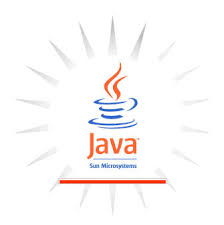String Comparison In Java -String Class Simplified in Java Part-2

0
18
• 1
•
•
•
•
•
•
•
•
•
1
ShareString Comparison is a very important part while dealing with strings. Due to this, I have created a separate post dedicated to String comparison. Below are the methods to compare the strings:

No Method Name Method Description Return Comment
1 compareTo Compares one string with other <0 if the String which is calling this method is lexicographically first(comes first in dictionary)</br>
=0 if the Strings are same</br>
>0 if the parameter passed is lexicographically first(comes first in dictionary)
This method is case sensative
2 compareToIgnoreCase Compares one string with other <0 if the String which is calling this method is lexicographically first(comes first in dictionary)</br>
=0 if the Strings are same</br>
>0 if the parameter passed is lexicographically first(comes first in dictionary)
This method is case insensative
``````
public class StringTest{
public static void main(String[] args)
{
String a="India";
String b="India";
String c="Indian";
String e="Vietnam";
String f="india";
System.out.println("When both are same, it generates "+ a.compareTo(b));
System.out.println("When both are not same, it generates "+ a.compareTo(c));
System.out.println("When both are not same, it generates "+ a.compareTo(d));
//lexicographically India is ahead of vietnam
System.out.println("When both are not same, it generates "+ a.compareTo(e));
//test of ignorecase
System.out.println("When both are not same case wise, it generates "+ a.compareToIgnoreCase(f));

}
}
``````

Output of the code:
\$javac StringTest.java
\$java -Xmx128M -Xms16M StringTest
When both are same, it generates 0
When both are not same, it generates -1
When both are not same, it generates 7
When both are not same, it generates -13
When both are not same case wise, it generates 0

The second set of String comparison methods: equals() and equalsIgnoreCase(). Both the methods are overridden method from class Object and compares character by character for the given two strings.

No Method Name Method Description Return Comment
1 equals() Checks if the two strings are equal boolean true or false
2 equalsIgnoreCase() Checks if the two strings are equal ignoring the case boolean true or false
``````

public class StringTest{
public static void main(String[] args)
{
String a="India";
String b="India";
String d="Indian";
String c=new String("India");
System.out.println("Checking equals with two same string gives "+a.equals(b));
System.out.println("Checking equals with two same string gives "+a.equals(d));
System.out.println("Checking equals with two same string gives "+a.equals(c));

}
}
``````

output of the code:
\$javac StringTest.java
\$java -Xmx128M -Xms16M StringTest
Checking equals with two same string gives true
Checking equals with two same string gives false
Checking equals with two same string gives true

The third method of comparing String is “==” operator.In Object class there is also a method calls equals() which checks the addresses instead of content.So if both string points to the String in String pool, or if both they are null, they will return true.Else JVM will call equals() method of String Class and they will return false.

``````
public class StringTest{
public static void main(String[] args)
{
String a="India";
String b="India";
String c=new String("India");
System.out.println("When both are same, it generates "+ (a==b));
System.out.println("When both are same, it generates "+ (a==c));

}
}``````

The output of the code:
\$javac StringTest.java
\$java -Xmx128M -Xms16M StringTest
When both are same, it generates true
When both are same, it generates false

Why we should not use == to compare a String?
Java has two types of storage for storing variable-Heap and Stack.While working with primitive datatypes,when we create variables of the primitive type it is stored in stack for quick access.
When we create an object that is String , it goes to heap. The address of the heap is given to the object(variable) stored to stack.It
So when == is applied to primitives to compare then it is ok. For objects(String), it is not applicable. It only checks if both the variable holds the same address.Otherwise it will always return falls. “==” operator check on the stack not on the heap.
you can refer to this link for all the documentation
So == checks for reference equality and equals() method check for content.

The explanation is given here also:
Author Credit: Ganesh
Johannes H

``````
String a="Test";
String b="Test";
if(a==b) ===> true
``````

This is because when you create any String literal, the JVM first searches for that literal in the String pool, and if it finds a match, that same reference will be given to the new String. Because of this, we get:

``````
(a==b) ===> true
``````

String Pool
b —————–> “test” <—————–a
However, == fails in the following case:

``````
String a="test";
String b=new String("test");
if (a==b) ===> false
``````

In this case for new String(“test”) the statement new String will be created on the heap, and that reference will be given to b, so b will be given a reference on the heap, not in String pool.

Now a is pointing to a String in the String pool while b is pointing to a String on the heap. Because of that we get:

if(a==b) ===> false.

String Pool
“test” <——————– a

Heap
“test” <——————– b
While .equals() always compares a value of String so it gives true in both cases:

``````
String a="Test";
String b="Test";
if(a.equals(b)) ===> true

String a="test";
String b=new String("test");
if(a.equals(b)) ===> true
``````

The last section of String comparison with Appache common Library:Appache provides a special called Utility that has the below methods for String comparison. It is little advanced version of String class’s comparison method. They are null safe.
The methods are:
1.equals() and equalsIgnoreCase() ==>Same as String’s equal() and equalsIgnoreCase()
2.equalsAny() and equalsAnyIgnoreCase()==> This is applicable for multiarg Scenarios. Returns true if both the values are same.
3.compare() and compareIgnoreCase()=>Null safe, hence two nulls are considered as true if compared.

Don't miss out!

Receive top technical news, lesson ideas, travel tips and more!

Give it a try. You can unsubscribe at any time.